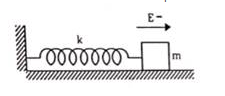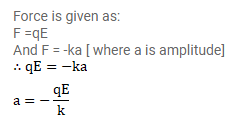# A block of mass $m$ and having a charge q is placedQuestion:

A block of mass $m$ and having a charge $q$ is placed on a smooth horizontal table and is connected to a wall through an unstressed spring of spring constant $k$ as shown in figure (29.E1). A horizontal electric field E parallel to the spring is switched on. Find the amplitude of the resulting SHM of the block.Solution: